Question

# Part A What volume of 1.75 M HCl in liters is needed to react completely (with...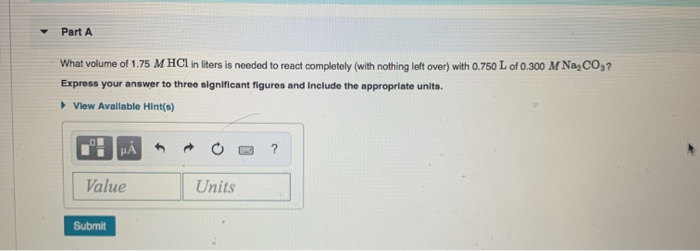Part A What volume of 1.75 M HCl in liters is needed to react completely (with nothing left over) with 0.750 L of 0.300 M NaCO3? Express your answer to three significant figures and include the appropriate units. View Available Hint(s) I HẢ * * 0 -3 ? Value Units Submit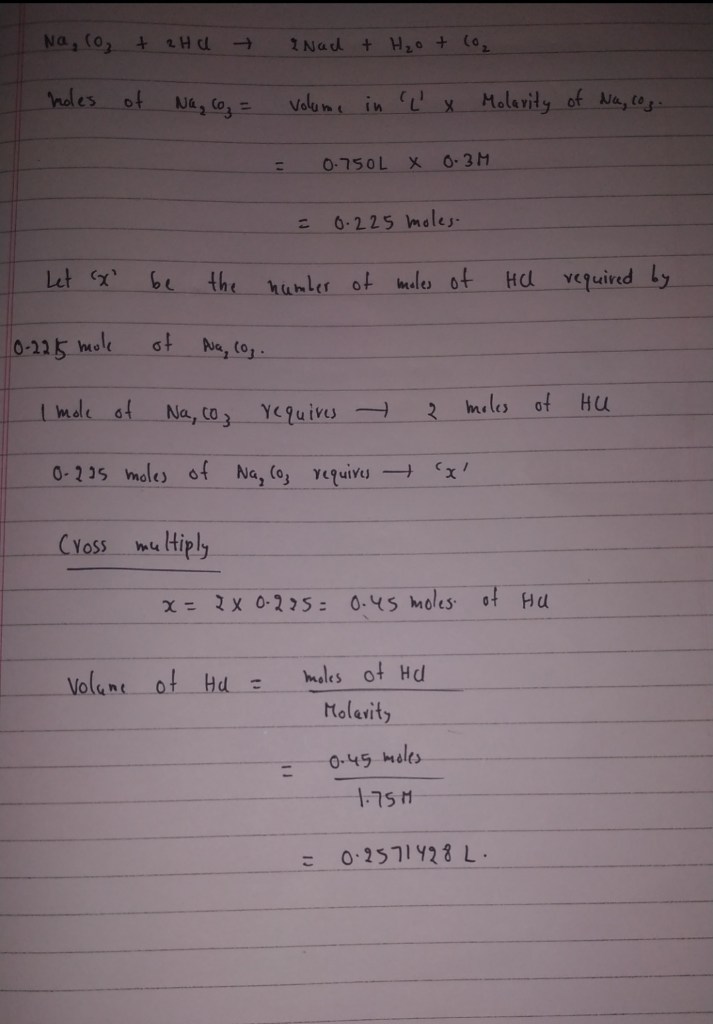#### Earn Coins

Coins can be redeemed for fabulous gifts.

Similar Homework Help Questions
• ### Part A What volume of 1.00 M HCl in liters is needed to react completely (with...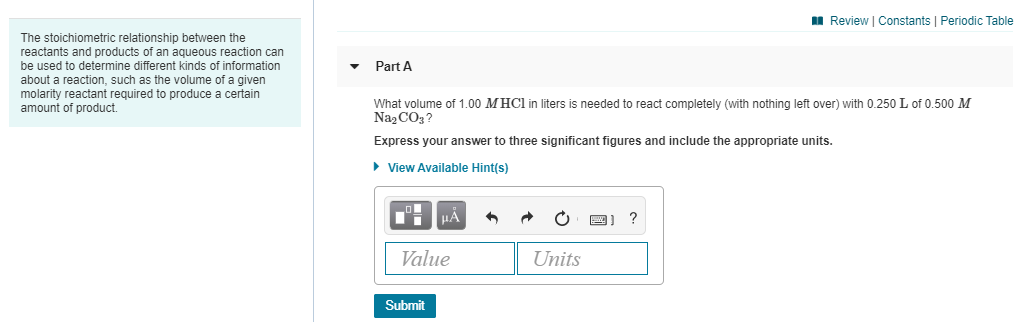Part A What volume of 1.00 M HCl in liters is needed to react completely (with nothing left over) with 0.250 L of 0.500 M Na2CO3? Express your answer to three significant figures and include the appropriate units. Part B A 419-mL sample of unknown HCl solution reacts completely with Na2CO3 to form 12.1 g CO2. What was the concentration of the HCl solution? Express your answer to three significant figures and include the appropriate units. A Review Constants Periodic...

• ### 1. What volume of 1.00 M HCl in liters is needed to react completely (with nothing...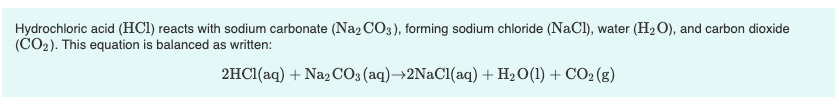1. What volume of 1.00 M HCl in liters is needed to react completely (with nothing left over) with 0.750 L of 0.200 M Na2CO3? Express your answer to three significant figures and include the appropriate units. 2. A 639-mL sample of unknown HCl solution reacts completely with Na2CO3 to form 15.1 g CO2. What was the concentration of the HCl solution? Express your answer to three significant figures and include the appropriate units. Hydrochloric acid (HCl) reacts with sodium...

• ### 1. What is the final volume in milliliters when 0.653 L of a 45.4 % (m/v)...

1. What is the final volume in milliliters when 0.653 L of a 45.4 % (m/v) solution is diluted to 24.0 % (m/v)? Express your answer with the appropriate units. 2. A 751 mL NaCl solution is diluted to a volume of 1.06 L and a concentration of 8.00 M . What was the initial concentration? Express your answer with the appropriate units. 3. What volume of 1.00 M HCl in liters is needed to react completely (with nothing left...

• ### The stoichiometric relationship between the reactants and products of an aqueous reaction can be used to...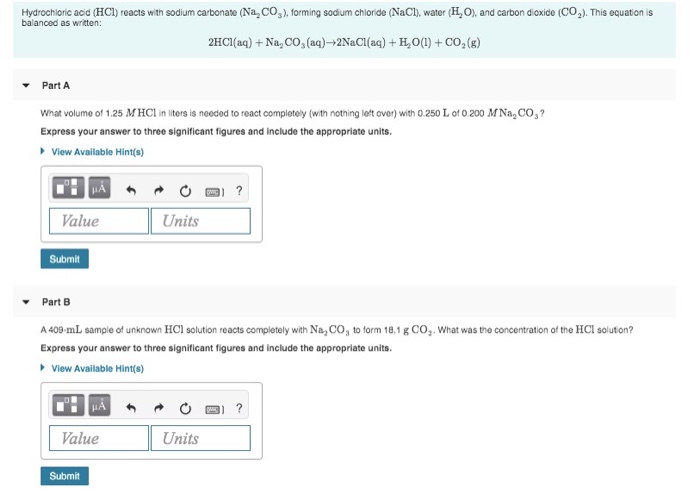The stoichiometric relationship between the reactants and products of an aqueous reaction can be used to determine different kinds of information about a reaction, such as the volume of a given molarity reactant required to produce a certain amount of product. Hydrochloric acid (HCl) reacts with sodium carbonate (Na CO2). forming sodium chloride (NaCl), water (HO), and carbon dioxide (C0.). This equation is balanced as written: 2HCl(aq) + Na, CO, (aq)-2NaCl(aq) + H,0(1) + CO2(g) Part A What volume of...

• ### The stoichiometric relationship between the reactants and products of an aqueous reaction can be used to...

The stoichiometric relationship between the reactants and products of an aqueous reaction can be used to determine different kinds of information about a reaction, such as the volume of a given molarity reactant required to produce a certain amount of product. Hydrochloric acid (HCl) reacts with sodium carbonate (Na2CO3), forming sodium chloride (NaCl), water (H2O), and carbon dioxide (CO2). This equation is balanced as written: 2HCl(aq)+Na2CO3(aq)→2NaCl(aq)+H2O(l)+CO2(g) a. What volume of 2.50 M HCl in liters is needed to react completely...

• ### 1.46 g H2 is allowed to react with 9.76 g N2, producing 1.75 g NH3 -...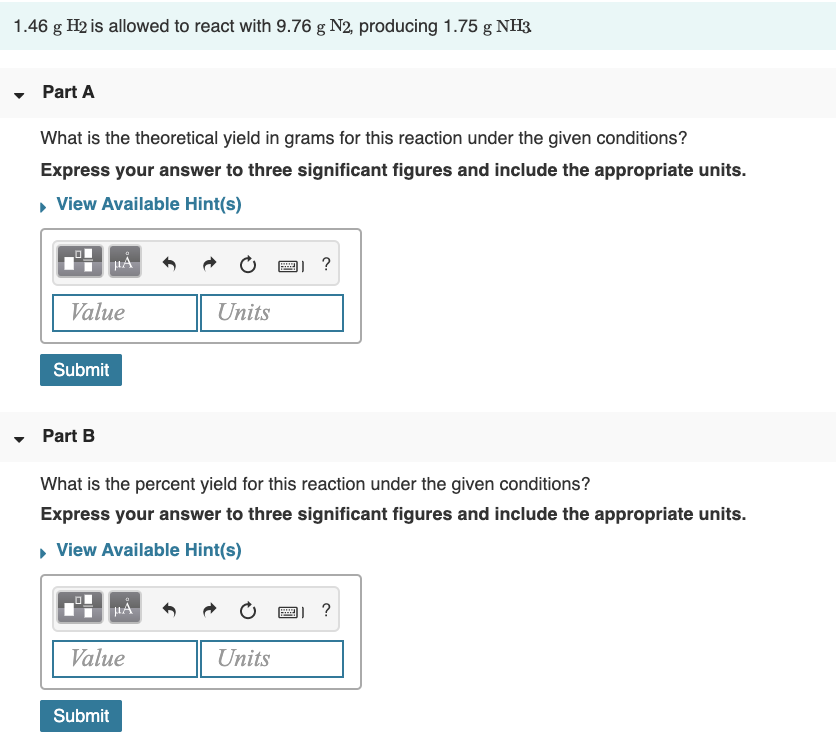1.46 g H2 is allowed to react with 9.76 g N2, producing 1.75 g NH3 - Part A What is the theoretical yield in grams for this reaction under the given conditions? Express your answer to three significant figures and include the appropriate units. View Available Hint(s) Value Units Submit Part B What is the percent yield for this reaction under the given conditions? Express your answer to three significant figures and include the appropriate units. View Available Hint(s) CPA...

• ### 9 of 28 A Review Constants | Periodic Table 2HCl(aq) + Na2CO3(aq) +2NaCl(aq) + H2O(l) +...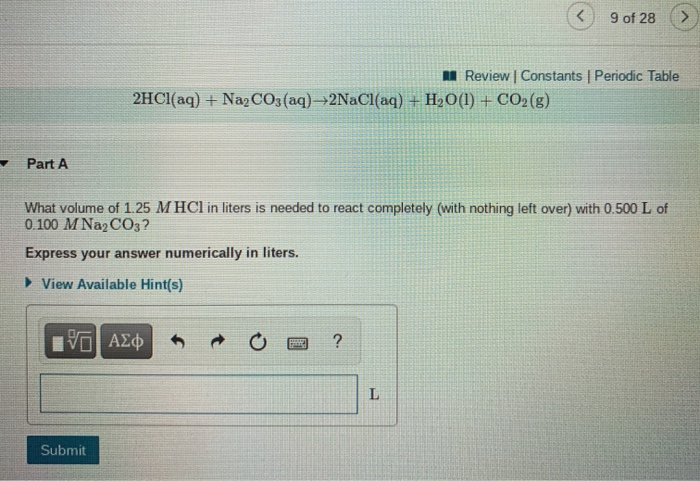9 of 28 A Review Constants | Periodic Table 2HCl(aq) + Na2CO3(aq) +2NaCl(aq) + H2O(l) + CO2(g) Part A What volume of 1.25 M HCl in liters is needed to react completely (with nothing left over) with 0.500 L of 0.100 M Na2CO3? Express your answer numerically in liters. ► View Available Hint(s) ΟΙ ΑΣΦ ? L Submit 9 of 28 Review | Constants Periodic Table Part B A 381-mL sample of unknown HCl solution reacts completely with Na2CO3 to...

• ### Part A What volume of 0.173 M Na3PO4 solution is necessary to completely react with 88.5...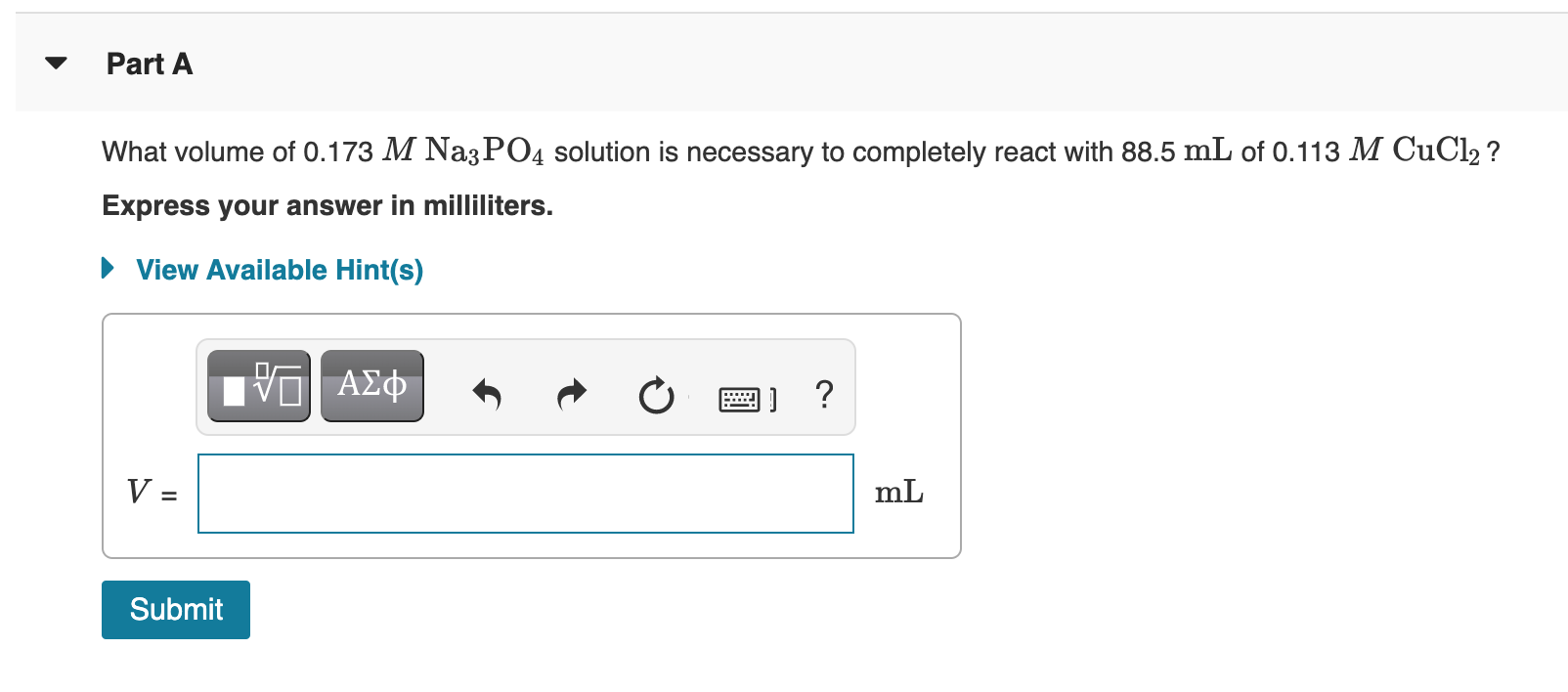Part A What volume of 0.173 M Na3PO4 solution is necessary to completely react with 88.5 mL of 0.113 M CuCl2? Express your answer in milliliters. ► View Available Hint(s) VO AXO 3. ? V = mL Submit

• ### Solution Stoichiometry Hydrochloric acid (HCl) reacts with sodium carbonate (Na2CO3), forming sodium chloride (NaCl), water (H2O),...

Solution Stoichiometry Hydrochloric acid (HCl) reacts with sodium carbonate (Na2CO3), forming sodium chloride (NaCl), water (H2O), and carbon dioxide (CO2). This equation is balanced as written: 2HCl(aq)+Na2CO3(aq)→2NaCl(aq)+H2O(l)+CO2(g) a) What volume of 2.75 M HCl in liters is needed to react completely (with nothing left over) with 0.750 L of 0.300 M Na2CO3? b) A 565-mL sample of unknown HCl solution reacts completely with Na2CO3 to form 10.1 g CO2. What was the concentration of the HCl solution? How do I...

• ### Answer: volume in L = 3. How many liters of 0.750 M HCl are needed to react with excess CaCO3 to produce 32.0 L of...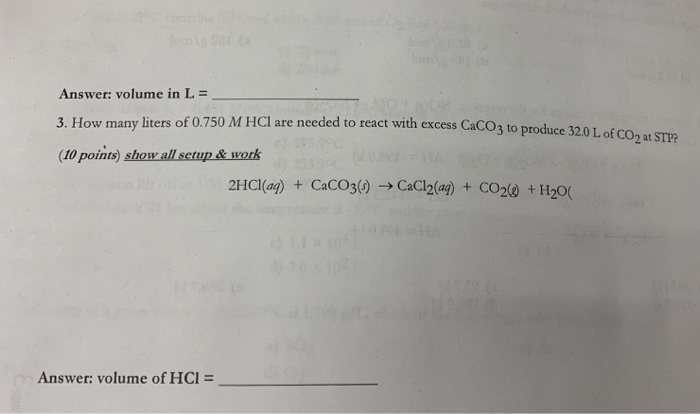Answer: volume in L = 3. How many liters of 0.750 M HCl are needed to react with excess CaCO3 to produce 32.0 L of CO2 at STP? (10 points) show all setup &work 2HCI(ag)+CaCO3) CaCl2(ag) + CO2@ +H20( Answer: volume of HCl =